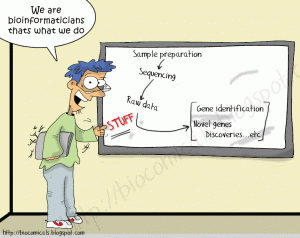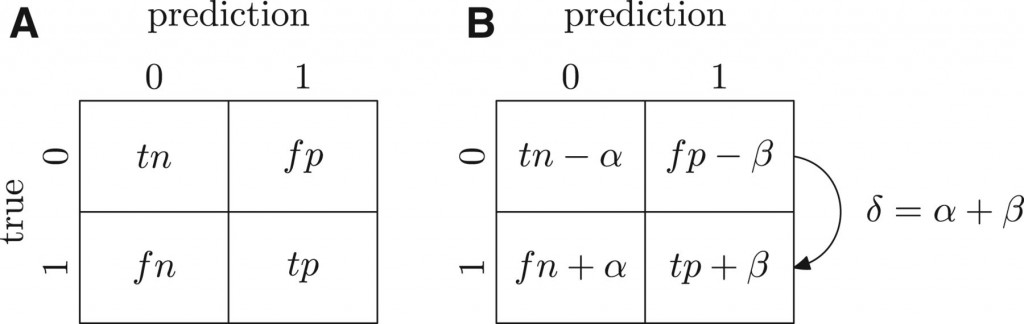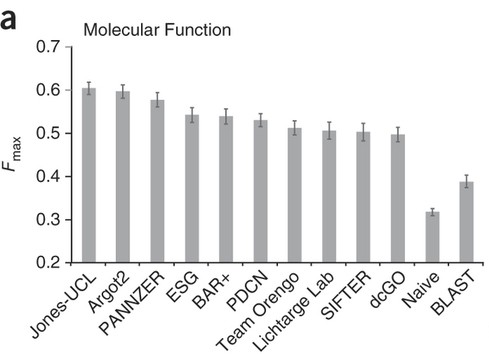## Estimating how much we don’t knowOr: “Estimating how much we don’t know, and how much it can hurt us”.

This post is about a paper I published recently with Predrag Radivojac’s group at Indiana University, who lead the study.

One of the main activities I’m involved with is CAFA,* the critical assessment of function annotations.   The general idea of CAFA is to assess how well protein function prediction algorithms work.

Why is it important to do that? Because today most of our understanding of what genes do comes from computational predictions, rather than actual experiments. For almost any given gene that is sequenced, its function is determined by putting its sequence through one or more function annotation algorithms. Computational annotation is cheaper and more feasible than cloning, translating, and assaying the gene product (typically a protein) to find out exactly what it does. Experiments can be long, expensive and,  in many cases, impossible to perform.But, by resorting to computational annotation of the function of proteins, we need to know how well can these algorithms actually perform. Enter CAFA, of which I have written before. CAFA is a community challenge that assesses the performance of  protein function prediction algorithms.

How does the CAFA challenge work? Well, briefly:

1. Target selection: we select a large number of proteins from SwissProt, UniProt-GOA and other databases. Those proteins have no experimental annotations, only computational ones. Those are the prediction targets.

2. Prediction phase: we publish the targets. Participating CAFA teams now have four months to provide their own functional annotations, using the Gene Ontology, a controlled vocabulary describing protein functions.

3. Growth phase: after four months, we close the predictions, and wait for another six months, or so. During those six months, some of the targets acquire experimentally-validated annotations. This typically means that biocurators have associated some of these proteins with papers for which experimental data have been provided, we call the targets that have been experimentally annotated during this phase benchmarks. Typically, the benchmarks are a small fraction of the targets. But since we pick about 100,000 targets, even a small fraction comprise a few hundred benchmarks, which is enough to assess how well programs match these proteins to their functions.

4. Assessment: we now use the benchmark proteins to assess how well the predictions are doing. We look at the GO terms assigned to the benchmarks, and compare them with the GO terms which the predictors gave.

Sounds good, what’s the problem then?

Well, there are a few, as there are with any methods challenge. For one, who’s to say that the metrics we use to assess algorithm prediction quality are the best ones? To address that, we actually use several metrics, but there are some we don’t use. Our AFP meetings always have very lively discussions about these metrics. No bloody noses yet, but pretty damn close.

Bu the issue I would like to talk about here is how much can we know at the time of the assessment? Suppose someone predicts that the target protein “X” is a kinase. Protein “X” happened to be experimentally annotated during the growth phase, so now it is a benchmark. However, it was not annotated as a kinase. So the prediction that “A” is a kinase is considered to be, at the assessment, a false positive. But is it really? Suppose that a year later, someone does discover that X is a kinase. The prediction was correct after all, but because we did not know ti at the time, we dinged that method a year ago when we shouldn’t have. The same goes for the converse: suppose “X” was not predicted to be a kinase, and was also assessed not to be a kinase. Two  years later, “X” is found out to be a kinase. In this case, not predicting a kinase function  for “X” was a false negative prediction. And we gave a free pass to the methods that did not catch that false negative. The figure blow illustrates that at time “A” we have an incomplete knowledge, when compared to a later time “B”.At the time of assessment (A), we claim to know all the false positives(fp) , false negatives (fn)  true positives (tp) and true negatives (tn) of the CAFA predictions. But then at a later time, (B), we may discover errors in our assessment as we gain more data. At time (B), α is the number of true negatives we did not know of at time (A), and β is the number of false positives we did not know of at time A. The sum of both errors is δ. Source: http://bioinformatics.oxfordjournals.org/content/30/17/i609.abstract

So how many of these false false-positives and false false-negatives are there? Putting it another way, by how much does the missing information at any given time affect our ability to accurately assess the accuracy of the function prediction algorithms? If we assess an algorithm today only to discover that are assessment is wrong a year from now, our assessment is not worth much, is it?

To answer this question, I first have to explain how we assess CAFA predictions. There are two chief ways we do that. First, there is a method using precision (pr) and recall (rc). Precision/recall assessments are quite common when assessing the performance of prediction programs.

$latex pr=\frac{tp}{tp+fp}&s=1$    $latex rc=\frac{tp}{tp+fn}&s=1$

The precision is the number of correct predictions out of all predictions, true and false. Recall is the number of correct predictions out of all known true annotations. (tp: true positives; fp: false positives; fn: false negatives). We can use the harmonic mean of precision and recall to boil them down to one number also known as F1:

$latex F_1 = 2\times \frac{pr\times rc}{pr+rc}&s=1$

The F1 is one metric we use to rank performance in CAFA. If a prediction is perfect, i.e. no false positives or false negatives, fp=0, fn=0 then the precision equals 1, the recall equals 1 and F1=1. On the other hand, if there are no true positives (tp=0 that is, the method didn’t get anything right), then F1 =0. between these two extremes lie the spectrum of  scores of methods predicting in CAFA. Here is how well the top-scoring methods did in CAFA1:These are the maximum F1 scores for all methods in the first CAFA. BLAST was used as a baseline, and is shown on the right. Taken from: http://www.nature.com/nmeth/journal/v10/n3/full/nmeth.2340.html

But the F1 (or rather Fmax, the maximum F1 for each method) given here is for time (A), when we first assess CAFA. But at time (A) our knowledge is incomplete. At time (B) we know more! We have some idea of the alpha and beta errors we made.(At later time points, C etc. we will know more still).

OK, so let’s rescore the methods at time B, call this new score F’1. First, the new precision and recall, pr‘ and rc‘ at time (B), when we are wiser than (or at least more knowledgeable of our errors at)  time (A).

$latex pr’=\frac{tp+\beta}{tp+fp}&s=1$      $latex rc’=\frac{tp+\beta}{tp+fn+\alpha+\beta}&s=1$

So F’1 is:

$latex F’_1= \frac{2\times tp+\beta}{2tp+fp+fn+\alpha+\beta}&s=1$

So what? Bear with me a bit longer.  We now formalized the F1 (at the time of our first assessment) and F’1 (at some later time when we know more and recognize our α  and  β errors). What we are interested in is whether the differences between F1 and F’1 are significant. We know that pr’pr because beta > 0. The change in precision is

$latex \Delta pr= pr’-pr= \frac{\beta}{tp+fp}$

So the precision can only grow, and the more false positives we discover to be true positives at time (B), the better our precision gets. OK, so when we deal with precision, having  missing information on false negatives at time (A) is bad once we have more knowledge at time (B).

But what about recall and missing information on false positives? After all, F1 is a product of both precision and recall.

With recall, the story is slightly different. When rc‘ is the recall on the new annotations.

$latex rc’=\frac{\beta}{\alpha+\beta}&s=1$

I won’t get into the details here, you can see the full derivation in the paper (or work it out for yourself), but the bottom line is:

$latex \Delta F_1 \begin{cases} \ge 0 & \text{if } rc’\ge \frac{1}{2}F_1 \\ <0 & \text{otherwise} \end{cases}&s=1$

This is a surprising finding because only if rc’ is greater than half of the F1 will the F’1 > F1 ( that is, ΔF1>0)
1 does not depend directly on precision, only on recall!

So the F1 measure is quite robust to changes for prediction tools operating in high precision but low recall areas, which is characteristic of many of the tools participating in CAFA. The study also shows that, on real protein data, changes in F1 are not that large over time.

To be fair, the other metric that we use in CAFA, semantic distance, is more sensitive to varying values of δ. But even then the error rate is low, and we can at estimate it using studies of predictions over previous years. Our study also has simulations, playing around with α and β values to see how much we can aggravate changes in F1 and semantic distance.

Bottom line: missing information will always give us some error in the way we assess function prediction programs. But we can at least estimate the extent of the error and, under realistic conditions, the error rate is acceptably low. Making decisions when you know you don’t have enough data is a toughie, and is a much-studied problem in machine learning and game theory. Here we quantified this problem for CAFA. At least in our case, what we don’t know can hurt us, but we can estimate the level of hurt (misassessing algorithm accuracy), and it doesn’t really hurt. Much.

Jiang, Y., Clark, W., Friedberg, I., & Radivojac, P. (2014). The impact of incomplete knowledge on the evaluation of protein function prediction: a structured-output learning perspective Bioinformatics, 30 (17) DOI: 10.1093/bioinformatics/btu472

(*For all you Hebrew speakers sniggering out there — yes, the acronym is, purposefully, the Hebrew slang for “slap” כאפה).